# High School Physics : Conservation of Momentum

## Example Questions

### Example Question #67 : Momentum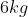ball is thrown west at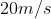and collides with a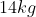ball while in the air. If the balls stick together in the crash and fall straight down to the ground, what was the velocity of the second ball?

Possible Answers: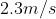east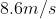east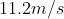downward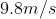west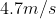east

Correct answer:east

Explanation:

We know that if the balls fell straight down after the crash, then the total momentum in the horizontal direction is zero. The only motion is due to gravity, rather than any remaining horizontal momentum. Based on conservation of momentum, the initial and final momentum values must be equal. If the final horizontal momentum is zero, then the initial horizontal momentum must also be zero.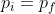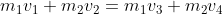In our situation, the final momentum is going to be zero.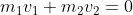Use the given values for the mass of each ball and initial velocity of the first ball to find the initial velocity of the second.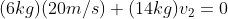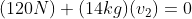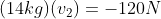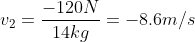The negative sign tells us the second ball is traveling in the opposite direction as the first, meaning it must be moving east.

### Example Question #68 : Momentum

Two equal mass balls (one green and the other yellow) are dropped from the same height and rebound off of the floor.  The yellow ball rebounds to a higher position.  Which ball is subjected to the greater magnitude of impulse during its collision with the floor?

Possible Answers:

The green ball

The yellow ball

Both balls were subjected to the same magnitude of impulse

It’s impossible to tell since the time interval and the force have not been provided in the problem

Correct answer:

The yellow ball

Explanation:

The impulse is equal to the change in momentum. The change in momentum is equal to the mass times the change in velocity.

The yellow ball rebounds higher and therefore has a higher velocity after the rebound.  Since it has a higher velocity after the collision, the overall change in momentum is greater.  Therefore since the change in momentum is greater, the impulse is higher.

### Example Question #69 : Momentum

A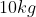ball moving at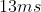strikes a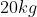ball at rest. After the collision theball is moving with a velocity of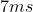. What is the velocity of the second ball?

Possible Answers: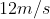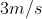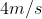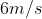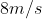Correct answer:Explanation:

This is an example of an elastic collision. We start with two masses and end with two masses with no loss of energy.

We can use the law of conservation of momentum to equate the initial and final terms.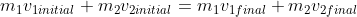Plug in the given values and solve for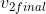.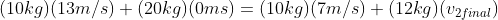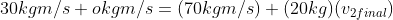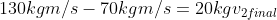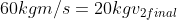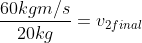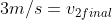### Example Question #70 : Momentum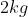object moves to the right at.  It collides head on with a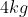object moving to the left at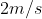.  Which statement is correct?

Possible Answers:

The total momentum before the collision is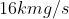and after the collision is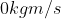The total momentum before and after the collision is zero.

The total momentum both before and after the collision is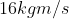None of the above is true

Correct answer:

The total momentum before and after the collision is zero.

Explanation:

The total momentum before the collision is equal to the momentum of each object added together.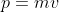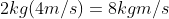Remember that moving to the left means that the object has a negative velocity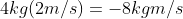Total momentum =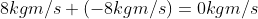According to the law of conservation of momentum, the total momentum at the end must equal the total momentum at the beginning.  Since the momentum at the beginning was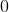, the momentum at the end would also be.

### All High School Physics Resources